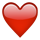## Forum Stats

• 3,751,622 Users
• 2,250,379 Discussions

Discussions

# Cannot delete using rownum why ??????

create table test(no int,name varchar2(25));

insert into test values(1,'aaaa');
insert into test values(2,'bbbb');
insert into test values(3,'cccc');
insert into test values(1,'dddd');
insert into test values(5,'aaaa');

delete from test where rownum=4;

0 rows deleted

i am unable to delete using rownum from the table pls help

Thanks
Rangan S
Tagged:

• Because the pseudo column rownum is dynamically assigned while fetching.
The database gets the first row from the table - rownum = 1, compares it to 4 and does not delete it.
The next row then is again assigned the rownum = 1, hence is also not deleted. And so on.

If you would try to delete rows with rownum < 3, the processing would be like this:
- first row, rownum = 1, compared to rownum < 3, gets deleted
- next row gets then rownum = 2, gets deleted
- next row gets rownum = 3 -> declined
- next row gets rownum = 3 -> declined
.
.
.

Rgds,
Guido
• Rownum doesn't work like that, is why!

A rownum is dynamically assigned for each row in the resultset - 1 for the first row, 2 for the second, etc.

When you say "where rownum < 3" oracle does this check in the where clause (nb. this is my interpretation of how it works, it may not be exactly how it works):

1. This the first row in the resultset so rownum = 1. Does that match the rownum < 3? Yup - I'll keep this row in the resultset
2. Next row - it's the second, so rownum = 2. Does that match the rownum? Yup - I'll keep this row in the resultset
3. Next row - it's the third, so rownum = 3. Does that match the rownum? Nope - discard the row from the resultset; I'll stop now.

And when you say "where rownum = 3":

1. This is the first row in the resultset so rownum = 1. Does that match rownum = 3? Nope - discard the row from the resultset. Try the next row
2. The next row is now the first in the resultset, so rownum = 1. Does that match rownum = 3? Nope - discard the row from the resultset. ....

Hopefully you can now see why saying "rownum = X" where X != 1 won't work

Edited by: Boneist on Sep 24, 2008 9:07 AM
For clarity

At least you can avoid such a figure!

Bye Alessandro
• If you will fire a query

select * from test where rownum=4;

it will return you 0 rows, so why while deleting you are getting a message saying 0 rows deleted.

instead you can fire a query

delete from test where rownum &lt;=4;

then it will delete 4 rows.
• Hi,